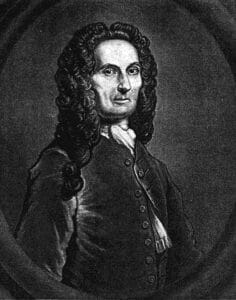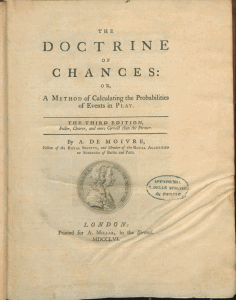# Abraham De Moivre: History, Biography, and AccomplishmentsAbraham de Moivre (1667–1754) was born in Vitry-Vitry-le-François, France. He was a passionate mathematician who made significant contributions to analytic geometry, trigonometry, and the theory of probability. Nonetheless, he is best known for the De Moivre Law (often referred to as the De Moivre’s Formula) and the Stirling’s Approximation.

Though Abraham de Moivre’s parents were Protestant, his father, Daniel de Moivre, was a surgeon, and hence, believed in the value of education. As a result, De Moivre first attended the Christian Brothers’ Catholic school in Vitry. At the age of eleven, his parents sent him to the Protestant Academy at Sedan.

Due to the intense Protestant persecution in 1682, the Protestant Academy at Sedan was suppressed. At this time, De Moivre enrolled to study logic at Saumur for two years. In 1684, he moved to Paris to continue his studies. However, this time around, he focused on the study of physics, and for the first time, had formal mathematics training.

As a Huguenot, he was pursued and sent to prison in 1685. After his release, he fled to England, where he spent the rest of his days in London. Here, he became close friends with Sir Isaac Newton, James Stirling, and Edmond Halley.

Although he worked mostly as a math tutor, De Moivre was elected fellow of the Royal Society of London in 1697 and a member of the Berlin and Paris academies.

### Other important accomplishments include the following:• The Doctrine of Chances, the first written and published book on probability theory (a branch of mathematics centered on the analysis of random phenomena).
• His works around Binet’s formula and the application of Fibonnaci’s “Golden Ratio.”
• The development of the central limit theorem, a key concept in probability theory.

Abraham De Moivre died on November 27th, 1754. Many of his papers were published after his death. Moreover, it is said that a big part of De Moivre’s work never saw the light of day, while others say they were published by different scholars of the time who claimed authorship of his developments.

## De Moivre Formula

In mathematics, the De Moivre’s formula (also known as De Moivre’s theorem) states that for any real number “x” and integer “n,” it holds that, where “i” is the imaginary unit, (i2 = −1).

(cos x + i sin x)n = cos(nx) + i sin(nx)

Its importance lies in the relationship it establishes between complex numbers and trigonometry.

By expanding (removing the parentheses) the left side of the equation and comparing the real and imaginary parts under the premise that “x” is real, it is possible to obtain useful expressions for cos(nx) and sin(nx).

The original formula does not work in non-integer powers “x,” but some generalizations and variations help to apply the same concept to different operations.

As a result, De Moivre’s theorem introduces a formula for computing powers of complex numbers.

## De Moivre’s Law

De Moivre’s law was first introduced in his 1725 book Annuities upon Lives. It is considered the first known example of an actuarial textbook. Despite its name, De Moivre did not consider his law to be an accurate description of the pattern of human mortality. In fact, he referred to it as a mere hypothesis and used it mainly as an effective approximation when calculating the cost of annuities.

In short, De Moivre’s Law is a simple law of mortality based on a linear survival function applied to a model.

S(x)=1−x/ω, 0 ≤x<ω

Its novelty relies on a single parameter called the ultimate age.

In actuarial notation (x) represents the status or life that has survived to age (x), and T(x) is the future lifetime of (x).

This law is applied today to discrete survival models known as life tables—which depict the probability of a person dying before his/her next birthday. In other words, it represents the survivorship of people from a defined population and can often be used to measure a population’s longevity.

## Other Contributions

Throughout his life, De Moivre published occasional papers on different branches of mathematics. Most of them offered solutions to somewhat fleeting problems in Newton’s calculus.

Nonetheless, in these lesser works, there is one trigonometric equation the discovery of which is sufficiently certain that it is still called De Moivre’s theorem:

(cos φi sin φ)n = cos nφ + i sin nφ

## Stirling’s Approximation

Stirling’s approximation, also known as Stirling’s formula, is an approximation for factorials leading to very accurate results.

### Stirling’s formula

James Stirling, a Scottish mathematician, began his scientific career at a time of significant political and religious conflicts. His formula is one of the decisive mathematical discoveries of the 18th century as it gives us an idea of the transformation of mathematics which took place in the seventeenth and eighteenth centuries. Although it is Stirling to whom it is attributed, the principle was genuinely developed by De Moivre.

(𝑛+12)log(𝑛)−𝑛+12log(2𝜋)

Abraham de Moivre first published the formula in 1730, in his book Miscellanea Analytica. He not only mentioned its almost definitive form but also demonstrated its usage. James Stirling published the same equation a few months later in his book Methodus Differentialis Sive Tractatus de Summatione et Interpolatione Serierum Infinitarum.

Stirling’s other relevant works include On the Figure of the Earth, and On the Variation of the Force of Gravity at Its Surface.

However, different from De Moivre, Stirling sets the value of c and improves the formula with the asymptotic development of five terms. Hence, the Wallis Integrals established the exact value of the constant.

The formula is used today in various areas, including statistical mechanics. Here, there are equations containing factorials of the number of particles. Since typical macroscopic systems have around N=1023 particles, Stirling’s formula is an excellent approximation.

Besides, Stirling’s formula is distinguishable, which allows a very approximate calculation of maximums and minimums in log factorial expressions in all kinds of calculations specially used in statistics and physics.

### Euler’s FormulaEuler’s formula, named after Leonhard Euler (a Swiss mathematician), is a mathematical formula which, much like De Moivre’s formula, establishes the fundamental relationship between the trigonometric functions and the complex exponential function.

Although it is based on some of the same principles as that explained by De Moivre’s theorem, it is considered by most scientists as a new and improved version. Even the well-known physicist Richard Feynman called Euler’s equation “the most remarkable formula in mathematics.”

Today, it is applied in many doctrines ranging from engineering to physics.

## Wrapping it up!

As you can see, Abraham De Moivre was an exceptional mathematician who made significant strides within math (and many other disciplines). As explained above, many of his formulas are still in use today.

As a result, De Moivre will always be remembered as of the most resilient mathematicians, despite having been incarcerated, judged by his immigrant status, and sometimes overlooked.